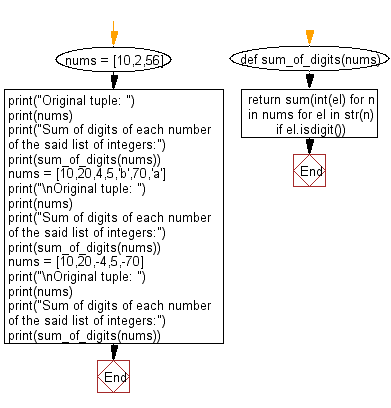﻿ Python: Compute the sum of digits of each number of a given list - w3resource# Python: Compute the sum of digits of each number of a given list

## Python List: Exercise - 146 with Solution

Write a Python program to compute the sum of digits of each number of a given list.

Sample Solution:

Python Code:

``````def sum_of_digits(nums):
return sum(int(el) for n in nums for el in str(n) if el.isdigit())

nums = [10,2,56]
print("Original tuple: ")
print(nums)
print("Sum of digits of each number of the said list of integers:")
print(sum_of_digits(nums))

nums = [10,20,4,5,'b',70,'a']
print("\nOriginal tuple: ")
print(nums)
print("Sum of digits of each number of the said list of integers:")
print(sum_of_digits(nums))

nums = [10,20,-4,5,-70]
print("\nOriginal tuple: ")
print(nums)
print("Sum of digits of each number of the said list of integers:")
print(sum_of_digits(nums))
```
```

Sample Output:

```Original tuple:
[10, 2, 56]
Sum of digits of each number of the said list of integers:
14

Original tuple:
[10, 20, 4, 5, 'b', 70, 'a']
Sum of digits of each number of the said list of integers:
19

Original tuple:
[10, 20, -4, 5, -70]
Sum of digits of each number of the said list of integers:
19
```

Flowchart:## Visualize Python code execution:

The following tool visualize what the computer is doing step-by-step as it executes the said program:

Python Code Editor:

Have another way to solve this solution? Contribute your code (and comments) through Disqus.

What is the difficulty level of this exercise?

Test your Python skills with w3resource's quiz

﻿

## Python: Tips of the Day

```print(2_000_000)
```2000000